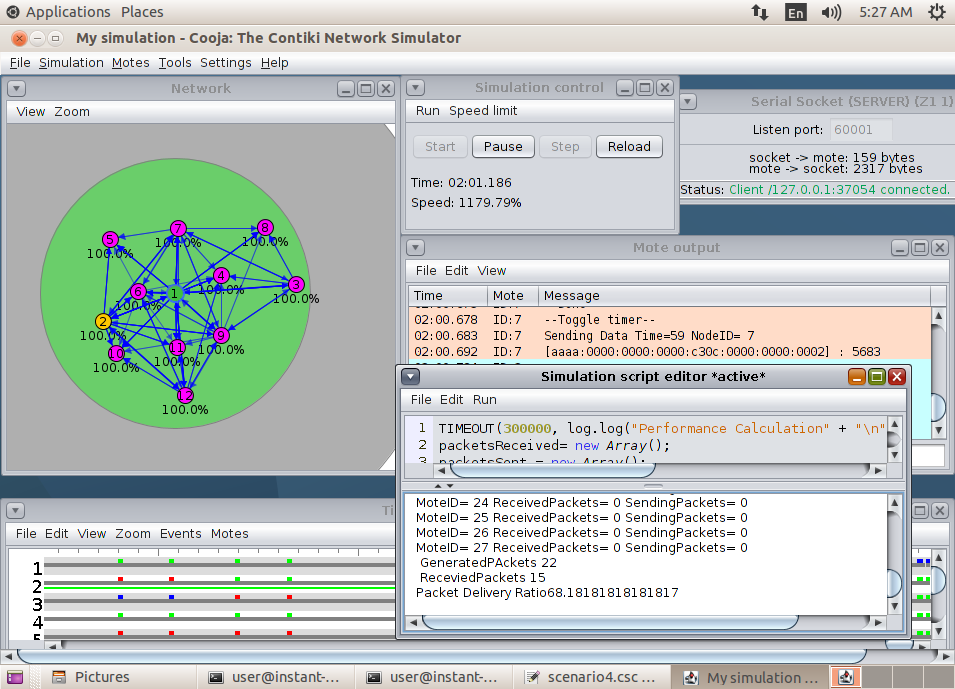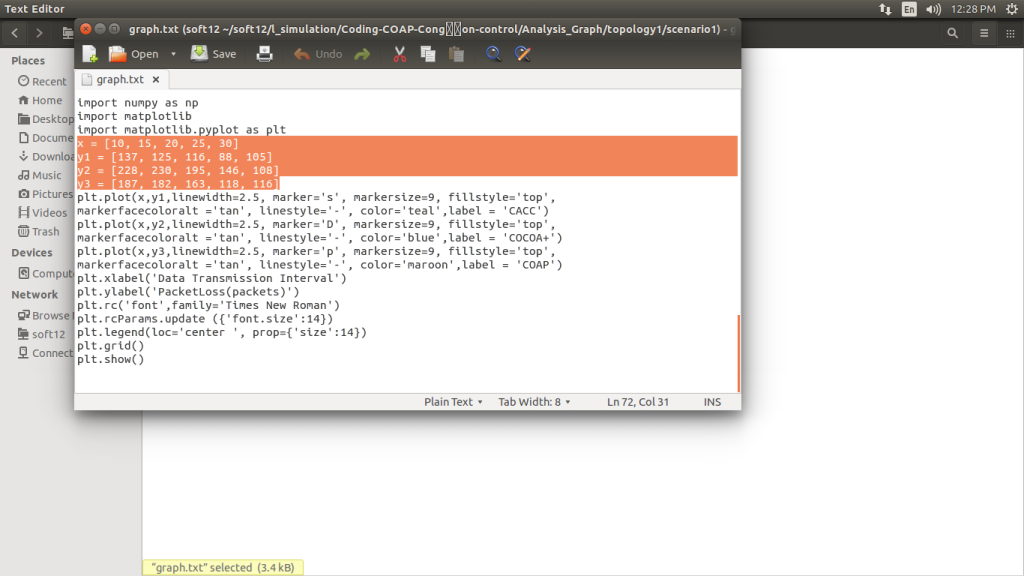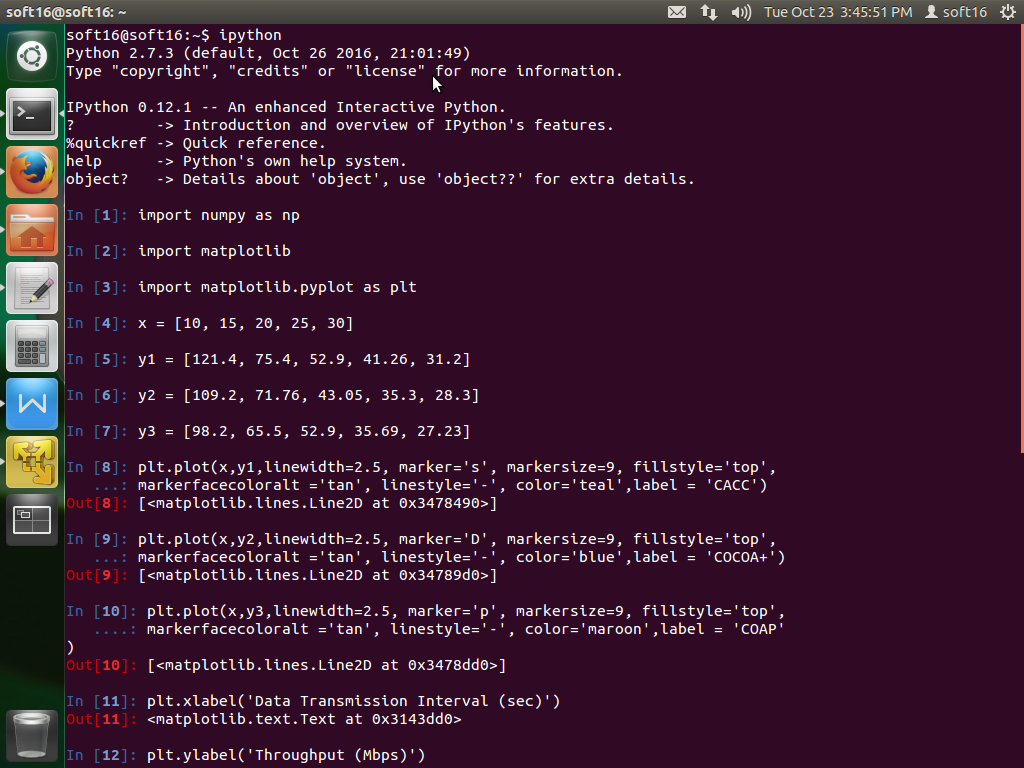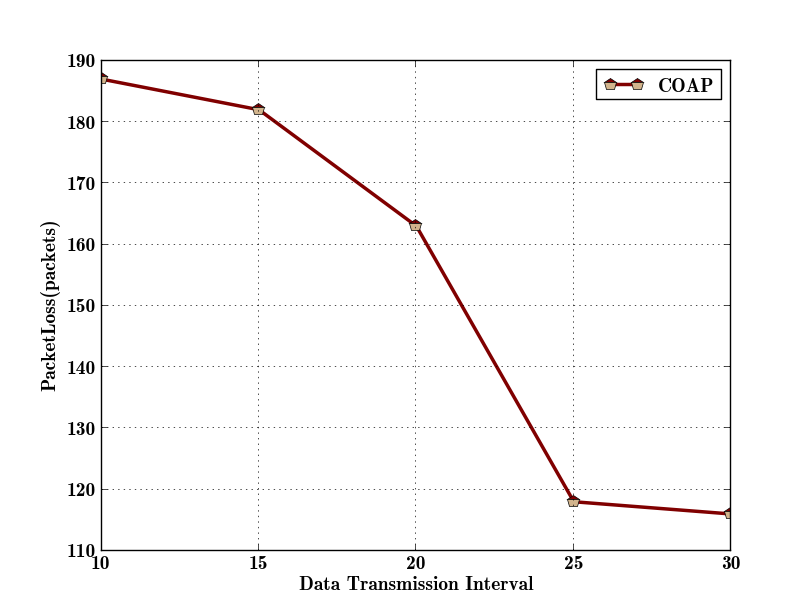• #5, First Floor, 4th Street Dr. Subbarayan Nagar Kodambakkam, Chennai-600 024 Landmark : Samiyar Madam
• pro@slogix.in
• +91- 81240 01111

#### Social List

##### How to calculate the Packet Delivery Ratio for CoAP message in cooja simulator?
###### Description

The packet delivery ratio is the ratio of packets successfully received to the total sent data packets.

###### Step-1

Border router location:
home/contiki/examples/ipv6/
rpl-border-router

motes >> create new motes type

Connect the router to server.
In terminal at above location.

make-connect-router-cooja

Start the simulation.

file in cooja network simulator.

After successful compilation
new terminal will be open.

(i.e).simulation script editor.

###### Open Cooja:

Edit script file,

After complete the simulation,
save it desired location (.csc)
file will be created.

into that file to calculate
metrics.

#### Code:

org.contikios.cooja.plugins.
ScriptRunner

< script>TIMEOUT(300000, log.log
("Performance Calculation" + "\n"));

packetsSent = new Array();

timeSent = new Array();

count = new Array();

nodeCount = 27;

data_length = 23;

senderID = 0;

PDR=0;

e_count = 0;

for(i = 3; i <= nodeCount; i++)

{

packetsSent[i] = 0;

timeSent[i] = 0;

}

while(1) {

YIELD();

msgArray = msg.split(' ');

if(msgArray.equals("Sending"))

{

if(msgArray.length == 5)

{

// sent packet

senderID = parseInt(msgArray);

packetsSent[senderID]++;

timeSent[senderID] = time;

}

}

if(msgArray.equals("Got"))

{

if(msgArray.length == 6)

{

{

}

totalclient=0;

for(i = 3; i <= nodeCount; i++)

{

totalclient++;

totalSent += packetsSent[i];

log.log("MoteID= " + i + "
" SendingPackets= " + packetsSent[i]
+ "\n");

}

log.log(" Generated Packets
" + totalSent + "\n");

* 100

log.log("Packet Delivery Ratio"
+ PDR + "\n");

}

}

}

Now reload the (.csc) file in
cooja network simulator.

After successful compilation
new terminal will be open.

(i.e).simulation script editor.

###### Step-3

Connect the server and
start the simulation.

It will display the packet
delivery ratio (PDR) in details.

>> Save the script editor
output . it calculates ,

i.Generated packets

iii.Packet Delivery Ratio###### Step-4

To plot the graph for
performance evaluation with collected
data packet information.

Matplotlip.pyplot
using this tool we can plot
the graph.

Open terminal >>enter
ipython>>enter following code.

#### Code:

import numpy as np

import matplotlib

import matplotlib.pyplot as plt

x = [10, 15, 20, 25, 30]

y1 = [137, 125, 116, 88, 105]

y2 = [228, 230, 195, 146, 108]

y3 = [187, 182, 163, 118, 116]

plt.plot(x,y1,linewidth=2.5,
marker='s', markersize=9,
fillstyle='top',

markerfacecoloralt ='tan',
linestyle='-', color='teal',
label = 'CACC')

plt.plot(x,y2,linewidth=2.5,
marker='D', markersize=9,
fillstyle='top',

markerfacecoloralt ='tan',
linestyle='-', color='blue'
,label = 'COCOA+')

plt.plot(x,y3,linewidth=2.5,
marker='p', markersize=9,
fillstyle='top',

markerfacecoloralt ='tan',
linestyle='-', color='maroon
',label = 'COAP')

plt.xlabel('Data Transmission
Interval')

plt.ylabel
('PacketLoss(packets)')

plt.rc('font',family=
'Times New Roman')

plt.rcParams.update
({'font.size':14})

plt.legend(loc='center
', prop={'size':14})

plt.grid()

plt.show()

data into your saved file before you
enter into plotting data.

In terminal,

Ipython >> enter codes>>enter###### Step-5

To create border router motes

home/user/contiki/examples/ipv6/
rpl-border-router/border-router.c

After compilation press enter
it will generate graph and save it.###### Output